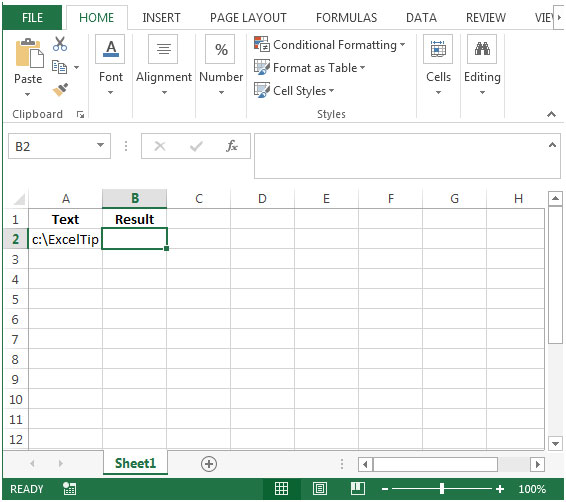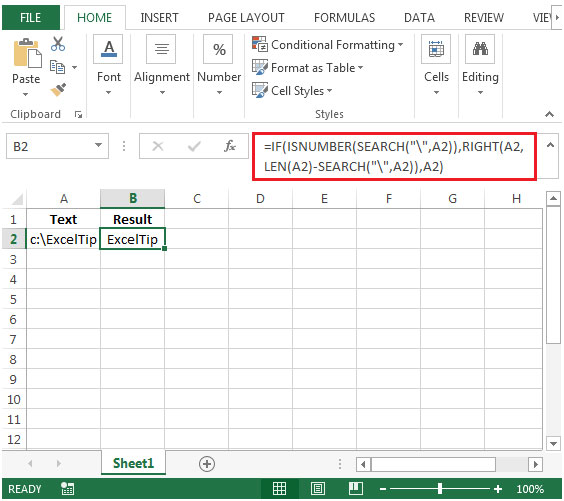# Parsing text to the right of a search criteria - delimiter in Microsoft Excel 2010

In this article we will learn how to find a formula to parsing text to the right of the search criteria, we will use a combination of "IF", "ISNUMBER", "SEARCH", "RIGHT" & "LEN" functions to get the output in Microsoft Excel 2010.

The IF function checks if a condition you specify is TRUE or FALSE. If the condition returns TRUE then it returns preset value, and if the condition returns FALSE then it returns another preset value.

Syntax = IF(logical_test,value_if_true,value_if_false)

logical_test: Logical test will test the condition or criteria.If condition meets then it returns the preset value, and if condition does not meet then it returnsanother preset value.

value_if_true: The value that you want to be returned if this argument returns TRUE.

value_if_false: The value that you want to be returned if this argument returns FALSE
ISNUMBER: Checks whether a value is a number, and returns TRUE or FALSE.

Syntax =ISNUMBER(value)

value: It is the value that you want to check whether it is a number or not.

SEARCH: Returns the number of the character at which a specific character or text string is first found, reading left to right (not case sensitive).

Syntax =SEARCH(find_text,within_text,start_num)

find_text: The text that you want to find.

within_text: It is the text in which you want to search within.

start_num: This is optional. It is the number in the string from which you want to extract data.
RIGHT: Returns the specified number of characters from the end of a text string.

Syntax: =RIGHT(text,num_chars)

text:It is the text string that contains character that you want to extract

num_chars:It specifies the number of characters from right you want to extract.
LEN: Returns the number of characters in a text string.

Syntax: =LEN(text)

text:It is the text whose length you want to find. It also countsthe spaces in between text.
Let us take an example:

• Cell A1 contains text as “c:\ExcelTip”.The output required is “ExcelTip” i.e. the text before “\” delimiter should not be shown in the result.• In cell B2, the formula would be
• =IF(ISNUMBER(SEARCH("\",A2)),RIGHT(A2,LEN(A2)-SEARCH("\",A2)),A2)
• Press Enter on your keyboard.• The function will return the result with out slash (/).

1.